### IMO Shortlist 2017 problem G4

Kvaliteta:
Avg: 0,0
Težina:
Avg: 7,0

In triangle$ABC$, let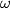$\omega$ be the excircle opposite to$A$. Let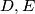$D, E$ and$F$ be the points where$\omega$ is tangent to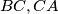$BC, CA$, and$AB$, respectively. The circle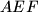$AEF$ intersects line$BC$ at$P$ and$Q$. Let$M$ be the midpoint of$AD$. Prove that the circle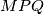$MPQ$ is tangent to$\omega$.

Izvor: https://www.imo-official.org/problems/IMO2017SL.pdf